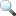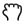## Zoom and rescale phase diagrams

In PandatTM, the diagrams are plotted from the table data in the workspace, and the data of the diagrams can be obtained through the corresponding table (How to plot a diagram from table data are descripted in detail in the table option page.) . Here, we will demonstrate how to zoom and rescale to see some parts of the diagram in more detail.

After you click the zoom iconin the toolbar, then hold the left button of the mouse and move the mouse to select a rectangular area on the graph to enlarge. The graph will zoom in to the selected area when the left button of the mouse is released. Double click the zoom icon will bring the zoom image back to the whole diagram again.

After you click the pan iconin the toolbar, the pan mode will be activated, when you put the cursor on the plot and roll the mouse wheel to enlarge or shrink the graph, keeping the current center of the graph unchanged. When your hold the left button of the mouse on the graph and move the mouse, the whole graph will move. Double click this button will bring the image back to the whole diagram again.

After you open and select a graph, the graph property window will be shown at the bottom left corner.  From this diagram property window, you can change your scale by setting the “Axis X min” and “Axis X min”;  “ Axis Y min” and “Axis Y max” under the group of “2 scale” .  You can also change other properties of the diagram, such as Title font and axis Line widthShow grid and ticks etc. Figure 1 shows the graph property window.

Figure 1. Graph property window

The procedure of rescale a triangle phase diagram: 1. set “Triangular plot” to “False”, 2. rescale the X and Y axis in Cartesian coordinate system with the same range, 3. set “Triangular plot” to “True” again to produce a rescaled triangle phase diagram.

In PandatTM, the ternary isotherm sections and liquidus projections are plotted as Gibbs triangle diagrams by default. The default scale is from 0 to 1 (or 0 to 100%). In those diagrams, the “Triangular Plot” is “True”.  When the Triangular plot is true, there is no option like “Axis X min”, which means you can not change the axis scale in the triangular manner.

Figure 2. Graph property window with Triangular Plot as True

If you want to rescale and only see part of this isothermal section as a triangle diagram. First, you should shift the “Triangular Plot” to “False”.   The triangle diagram will change to Cartesian coordinate diagram. Now you can change the minimum and maximum values of the X axis and Y axis. Figure 3 shows the above ternary phase diagram rescaled as 0-20% Zn for X axis and 0-20% Al for Y axis.

Figure 3. Change to cartesian coordinate diagram to rescale the phase diagram

If the X axis and Y axis are set as the same range, then you can change the “Triangular Plot” to “True” again, the rescaled triangle phase diagram is shown as Figure 4.

Figure 4. Triangular plot at Mg rich corner after rescale

If the X axis and Y axis have different range, a warning message showing as “Triangle Plot must be in equal range for X axis and Y axis” will appear, as shown in Figure 5. (Here we set X axis 0-20% and Y axis as 0-30%).

Figure 5. Error message shows the X axis and Y axis has different range and can not plot triangle diagram